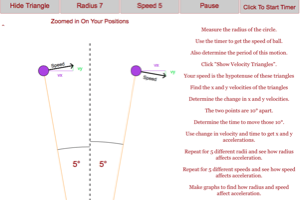Information About The Program
Circular Acceleration (Tool)This program gives students a visual representation of why an object moving in a circle at constant speed is accelerating. This program will guide students through a method of calculating the circular acceleration based on the change in velocity at two nearby positions. Students will be able to change radius and speed to see how each factor affects the circular acceleration.
Below are any Resources that go with this program